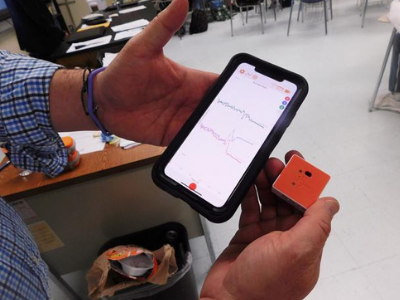Does a falling object have potential energy or kinetic energy or both? In other potential energy experiments, we demonstrated the Law of Conservation of Energy: energy can neither be created nor destroyed, but instead, energy transfers from one form to another. In this investigation, we will take a look at the role of gravity in energy transfer. This investigation aligns with NGSS MS-PS3-1 and MS-PS3-5 and can be scaled up for high school students to address HS-PS3-1 and HS-PS3-2.

TEACHERS: Find the assignable, interactive version of this lesson in PocketLab Notebook here.

The disciplinary core idea behind this standard is PS2.A: Definitions of Energy. It specifically looks at energy in motion (kinetic energy) and its relationship with the mass of the object in motion and the speed of the object. In the setup of the lab, students use a cart traveling down to test how changing the cart's changing speed will affect its kinetic energy and how changing the mass of the cart will change its kinetic energy. A PocketLab Voyager is placed on either the wheel of the cart or directly on top of the cart, in order to measure its speed as it travels down a ramp.

At different points down the ramp, students calculate the gravitational potential energy and the kinetic energy of the cart. In their calculations they will understand the relationship between GPE and KE, helping them with a deeper understanding of concepts in energy conservation, Crosscutting Concepts, Scale Proportion, and Quantity. In the crosscutting concepts (specifically how mass and velocity relate to kinetic energy and how mass and height relate to gravitational potential energy). For every run, students must use the Science and Engineering Practice, Analyzing and Interpreting Data, to process how the changing variables affect the collected velocity data and the calculated kinetic energy data. In the data analysis, students must also show in tables and graphs how the potential and kinetic energy changes in relation to the distance the cart has traveled, the speed of the cart, and the mass of the cart.

MS-PS2-2: Construct and interpret graphical displays of data to describe the relationship between kinetic energy to the mass of an object and the speed of the object.

The standard is broken down into the three NGSS pillars below:
Science and Engineering Practices - Analyzing and Interpreting Data
Disciplinary Core Ideas - PS3.A Definitions of Energy
Crosscutting Concepts - Scale, Proportion, and Quantity

#### Introduction

Potential energy is energy that is stored in an object. Potential energy can transfer into other forms of energy like kinetic energy. Kinetic energy is the energy an object has because of its motion.

A ball held in the air, for example, has gravitational potential energy. If released, as the ball moves faster and faster toward the ground, the force of gravity will transfer the potential energy to kinetic energy. The higher the ball, the more gravitational potential energy -- it will fall longer and faster as it accelerates toward the earth.

Now, if two balls are dropped from the same height, but one has more mass, which ball will require more energy to stop? Which ball, therefore, has more kinetic energy?

We can prove this with a science experiment using your PocketLab.

#### Objective

In this experiment, students will:

1. Gather evidence and data to support the Law of Conservation of Energy.
2. Collect data to calculate the amount of energy in a system at different moments.
3. Determine how the total energy in a system is broken up into different types of energy at different moments and how that is related to the law of conservation of energy.
4. Determine how the mass of a cart affects its gravitational potential energy and kinetic energy as it rolls down a ramp.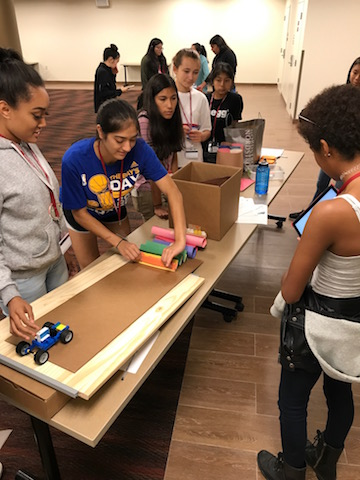#### Materials

1. Sensor: PocketLab G-Force, or PocketLab Voyager
2. Cart: PocketLab HotRodcart kitphysics cart, Hot Wheels car, or any other small toy car
4. Mass set
5. Scale
6. Measuring tape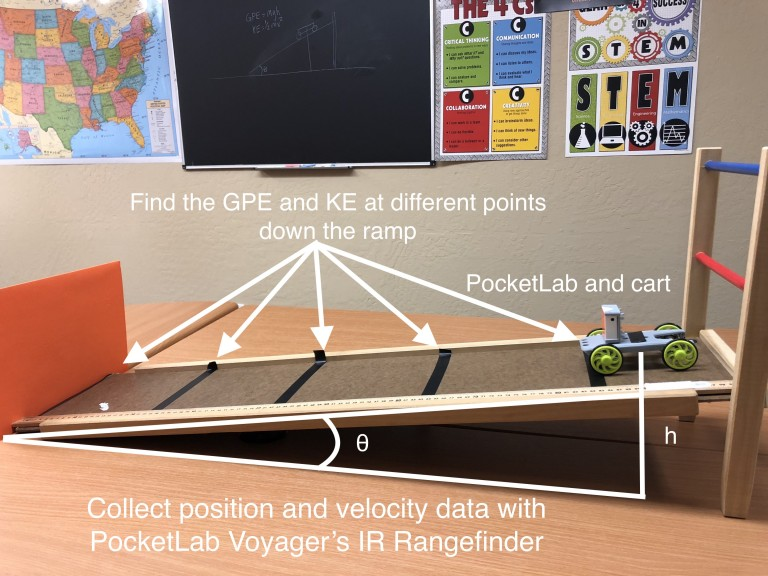#### Formulas and Keywords

Define the following:

• Gravitational Potential Energy (GPE)
• Kinetic Energy (KE)
• Velocity (v)
• Law of Conservation of Energy
• Total Energy (TE)
• Thermal Energy (Therm E)
• Friction

Formulas for lab:

GPE = mghKE = ½ mv^2

#### Hypothesis

Write a prediction to answer the following question: If the mass of the cart is increased, how will the cart’s GPE, KE, TE, and Velocity be affected as the cart rolls down the ramp?  Explain your hypothesis with either background knowledge about energy, forces, and motion and/or information gathered in the introduction and diagrams below.

#### Procedure

To measure position and velocity, use either the VelocityLab App (PocketLab One or PocketLab Voyager) or the infrared rangefinder sensor (PocketLab Voyager only).

Infrared Rangefinder (PocketLab Voyager)

• Go to the PocketLab Web App (in a Chrome browser) using the following address: thepocketlab.com/app or open up the PocketLab mobile app.
• For instruction on how to use the PocketLab Web App go here.
• Click on the "Change Graph" icon. Click "Rangefinder" and "Rangefinder Velocity".
• When setting up your cart and ramp, you'll need to make sure the rangefinder has a "wall" (a cardboard box will do) at the bottom of the ramp to give the rangefinder a clear signal.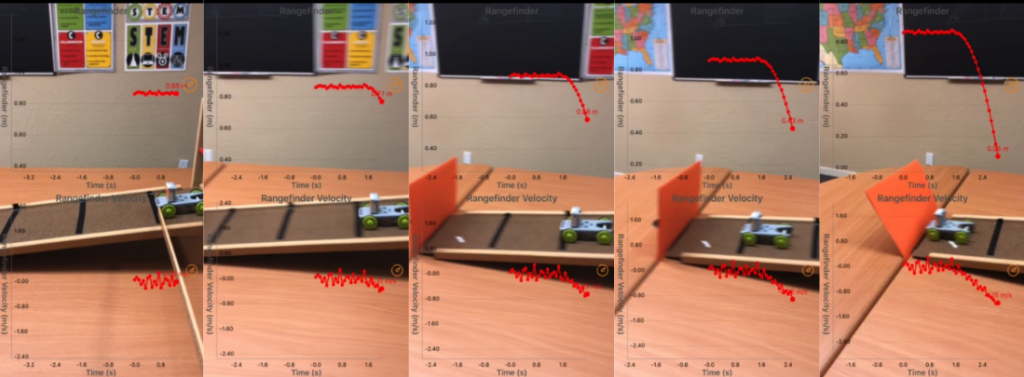Example of PocketLab Voyager on cart measuring position and velocity with IR Rangefinder at different points down the ramp

VelocityLab App (PocketLab Voyager or PocketLab One)

• Go to the VelocityLab Web App (in a Chrome browser) using the following address: thepocketlab.com/app and on the first menu click on the "Connect to VelocityLab" button. On iOS, use the VelocityLab app available in the App Store
• Turn on the PocketLab Voyager by clicking the button on the top.
• For instruction on how to use the PocketLab Web App go here
• Follow the prompts on the VelocityLab setup wizard.
• Click on the "Change Graph" icon. Click to view "Position" and "Velocity".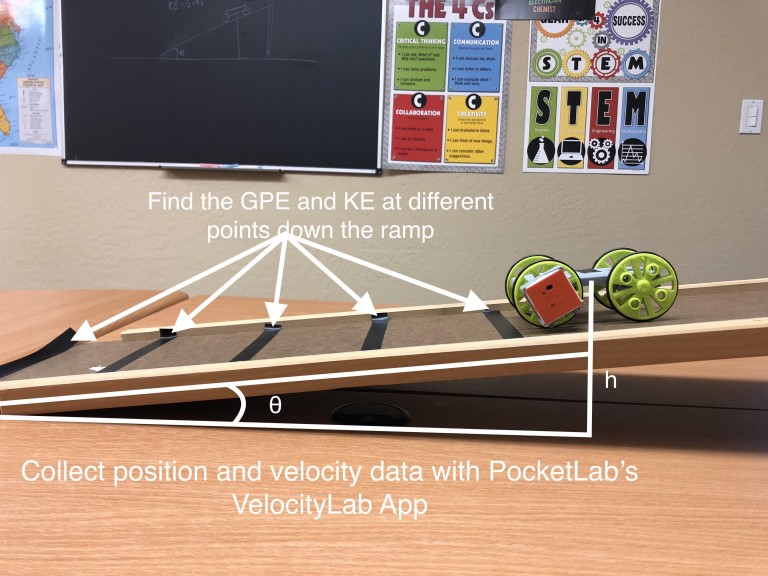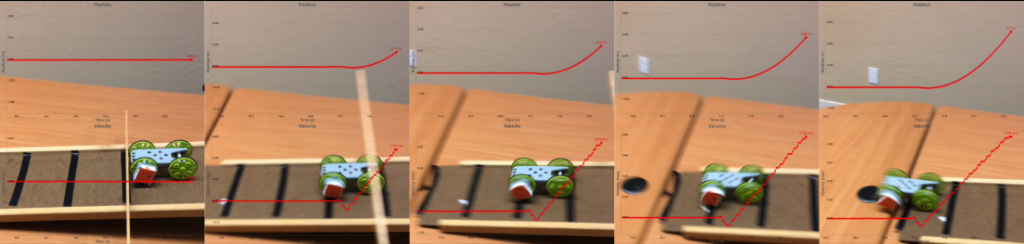Example of PocketLab on cart measuring position and velocity at different points down a ramp with PocketLab's VelocityLab App.

#### Collecting Evidence (Data and Observation)

Control Variables for Every Run

For every new run, you will change the independent variable but keep a set of control variables the same. This is to make sure the experiment is accurate. Record these control variables below:

Control Variables

 Angle of ramp Height of cart at top of ramp Distance cart will travel down the ramp

Independent Variables for Every Run
For every run you will be testing an independent variable. You will change the value o this variable to see how the change affects your dependent variables, measured in your data and seen in your observations.

 Independent Variable Mass of Cart

Data Collection for Run 1
Find the mass of the cart with the PocketLab attached and record it as the value of your Independent Variable for Run 1.

Independent Variable

 Mass of Cart
 Run 1 Observations:

• Set up the cart at the top of the ramp according to your control variables
• Begin recording data for Trial 1.
• Roll the cart down the ramp.
• Use the data to fill out the following table for Trial 1
• Repeat steps for Trials 2 and 3.
• Record any overall observations in the Run 1: Observations section above.

Run 1 - Trial 1

 Height of cart (m) Displacement (m) Velocity (m/s) GPE (J) KE (J)

Run 1 - Trial 2

 Height of cart (m) Displacement (m) Velocity (m/s) GPE (J) KE (J)

Run 1 - Trial 3

 Height of cart (m) Displacement (m) Velocity (m/s) GPE (J) KE (J)

Data Collection for Run 2

Add a mass from your mass set to the cart. Find the new mass of the cart with PocketLab and added mass and record it as the value of your Independent Variable for Run 2.

Independent Variable

 Mass of Cart
 Run 2 Observations

• Set up the cart at the top of the ramp according to your control variables
• Begin recording data for Trial 1.
• Roll the cart down the ramp.
• Use the data to fill out the following table for Trial 1
• Repeat steps for Trials 2 and 3.
• Record any overall observations in the Run 2: Observations section above.

Run 2 - Trial 1

 Height of cart (m) Displacement (m) Velocity (m/s) GPE (J) KE (J)

Run 2 - Trial 2

 Height of cart (m) Displacement (m) Velocity (m/s) GPE (J) KE (J)

Run 2 - Trial 3

 Height of cart (m) Displacement (m) Velocity (m/s) GPE (J) KE (J)

Data Collection for Run 3

Add a mass from your mass set to the cart. Find the new mass of the cart with PocketLab and added mass and record it as the value of your Independent Variable for Run 3.

Independent Variable

 Mass of Cart
 Run 3 Observations

• Set up the cart at the top of the ramp according to your control variables
• Begin recording data for Trial 1.
• Roll the cart down the ramp.-Use the data to fill out the following table for Trial 1
• Repeat steps for Trials 2 and 3.
• Record any overall observations in the Run 3: Observations section above.

Run 3 - Trial 1

 Height of cart (m) Displacement (m) Velocity (m/s) GPE (J) KE (J)

Run 3 - Trial 2

 Height of cart (m) Displacement (m) Velocity (m/s) GPE (J) KE (J)

Run 3 - Trial 3

 Height of cart (m) Displacement (m) Velocity (m/s) GPE (J) KE (J)

#### Data Analysis

Average the trials from each run below. Leave the bottom two rows of each table empty for now.

Run 1 Average

 Height of cart (m) Displacement (m) Velocity (m/s) GPE (J) KE (J)

Run 2 Average

 Height of cart (m) Displacement (m) Velocity (m/s) GPE (J) KE (J)

Run 3 Average

 Height of cart (m) Displacement (m) Velocity (m/s) GPE (J) KE (J)

#### Data Analysis Questions

• Look at the average data for Run 1. Add the GPE and KE at every location. Is the total energy of the system equal to just the GPE plus the KE? If that is the case, what is happening to the total energy in the system? Does this match the Law of Conservation of Energy? Explain.
• Something is missing in this data model. Look back at the formulas and keywords. Explain what is missing and how it relates to the Law of Conservation of Energy.
• Using the averages for each run, find the missing data and add it to the data tables above. Now find the total energy (TE) in the system at each point and add it to the data tables also. Use the empty rows to add the new data.
• Draw a series of bar graphs for each Run Average showing the distribution of energy and total energy at each location on the ramp.
• Looking at the bar graphs, what pattern do you notice?
• Did the average velocity for each location change much between the runs?

#### Conclusion and Lab Report

Option 1: Write a concluding paragraph that answers the Conclusion Questions at the bottom of the page.
Option 2: Write a full lab report for this lab activity. A lab report is a great way to summarize how you conducted your experiment and tested your hypothesis, the data collected, and any conclusions you can draw about the scientific question that was tested. In your lab report include:

1. Your original hypothesis from the beginning of the lab (in this case a description of your sketches).
2. The objectives or scientific questions you wanted to answer with the lab activity
3. What materials you used in the experiment.
4. A detailed description of how the lab was set up and how you tested your hypothesis.
7. A conclusion that answers the Conclusion Questions below.

#### Conclusion Questions

• Look back at your hypothesis from the beginning of the lab. Was your hypothesis valid or invalid? Why or why not? Support your answer with data/evidence collected from the lab experiment and scientific reasoning about energy, forces, and motion.
• GPE: Describe the relationship between an object’s height and its gravitational potential energy? Did you see evidence of this relationship in the data collected? Explain.
• GPE: Describe the relationship between an object’s mass and its gravitational potential energy? Did you see evidence of this relationship in the data collected? Explain.
• KE: Describe the relationship between an object’s mass and its kinetic energy? Did you see evidence of this relationship in the data collected? Explain.
• KE: Describe the relationship between an object’s velocity and its kinetic energy? Did you see evidence of this relationship in the data collected? Explain.
• Velocity: In today’s scenario, describe the relationship between an object’s mass and its velocity? Did you see evidence of this relationship in the data collected? Explain.
• Velocity and KE: Think about a large bus and a small car traveling at the same velocity. Which vehicle can do more damage in a crash? Explain your answer using data collected in today’s lab activity.
• Thermal Energy: Rub your hands together slowly for 10 seconds and then place your palms on your face. Rub your hands together quickly for 10 seconds and place your palms on your face. Do you feel a difference in thermal energy? How does this relate to the kinetic energy of the cart and the thermal energy produced in the system? Explain.
• Thinking especially about the bar graphs drawn in the data analysis section, did the data/evidence collected and observations made during the lab activity support the law of conservation of energy? Explain.

TEACHERS: Find the assignable, interactive version of this lesson in PocketLab Notebook here.

# Example Student Lab Report and Data

#### Hypothesis

If the mass of the cart increases, I predict the cart's GPE, KE, TE, and Velocity will all increase. I believe the added mass will make the cart go faster down the ramp which will also cause the KE of the cart to increase because KE = 1/2 mv^2. I believe the GPE will increase because the equation for GPE (GPE = mgh) shows that increasing either the mass or the height of the object will increase the GPE. I believe the TE of the cart will increase because both the GPE and KE will increase.

#### Objectives

1. Gather evidence and data to support the Law of Conservation of Energy.
2. Collect data to calculate the amount of energy in a system at different moments.
3. Determine how the total energy in a system is broken up into different types of energy at different moments and how that is related to the Law of Conservation of Energy.
4. Determine how the mass of a cart affects its gravitational potential energy and kinetic energy as it rolls down a ramp.

#### Materials

1. PocketLab Voyager
2. PocketLab HotRod
3. Ramp
4. Mass set
5. Scale
6. Measuring tape

#### Description

Using PocketLab Voyager and the VelocityLab App, we ran the cart down the ramp starting .50 m up the ramp. The height of the cart (measured directly from the ground) at .50 m up the ramp was 0.12 m. One-quarter of the way down the ramp, the height of the cart was 0.08 m, 1/2 down the ramp it was .06 m, 3/4 of the way down the ramp it was 0.03m, and all the way down the ramp was 0.0 m. This data, along with the mass of the cart was used to calculate the GPE of the cart at different locations. Using the Position and Velocity data from VelocityLab, we found the velocity of the cart 1/4, 1/2, 3/4, and all the way down the ramp. This was used to calculate the KE of the cart. We conducted three runs for this experiment. At each run, we increased the mass of the cart to see how it affected the GPE, KE, Velocity, and TE of the cart. For each run, we conducted three trials and averaged our results. For our "Resultant Visualization" we compared the increase in the cart's mass to the average KE of the cart at the bottom of the ramp for each run.

#### Collecting Evidence: Data and Observations

Run 1

 Independent Variable
 Mass of Cart 0.176kg
 Run 1 Observations The velocity data 1/4, 1/2, 3/4, and all the way down the ramp were fairly consistent across the three trials. The GPE of the cart was the same across trials. The KE of the cart was also consistent across trials. The resultant data listed here is the average top KE at the bottom of the ramp across all three trials. Resultant for Data Visualizations: KE at bottom of ramp = 0.071 J

Run 1 Trial 3 (Velocity at bottom of ramp = 0.90 m/s)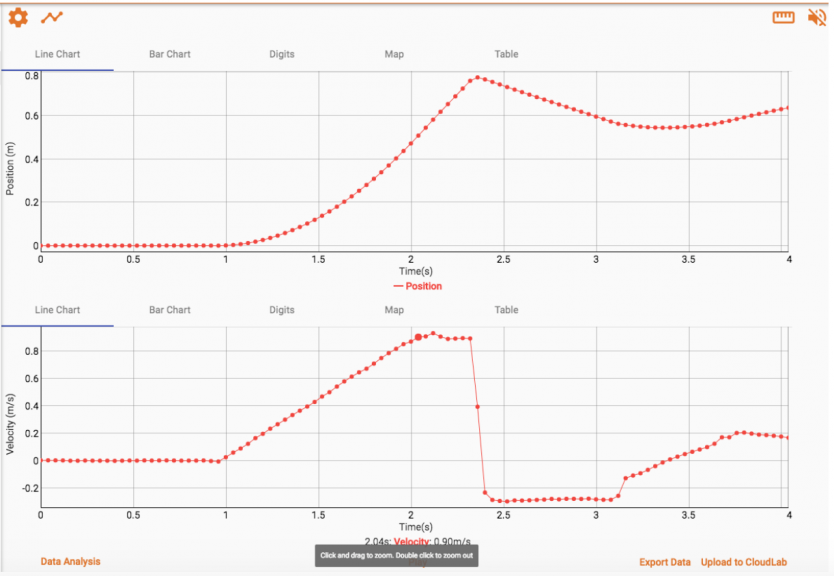Run 2

 Independent Variable
 Mass of Cart 0.215 kg
 Run 2 Observations As the cart traveled down the ramp, we noticed that the velocities at different points didn't change much from the data in Trial 1. The added mass didn't seem to have an effect on the velocity of the cart as it went down the ramp. The Kinetic Energy calculated increased, but that seemed to be a result of the increasing mass in the equation, not an increase in Velocity. The resultant shows the average Kinetic Energy of the cart at the bottom of the ramp. Resultant for Data Visualization: KE at bottom of ramp = 0.092 J

Run 2 Trial 3 (Velocity at bottom of ramp = 0.94 m/s)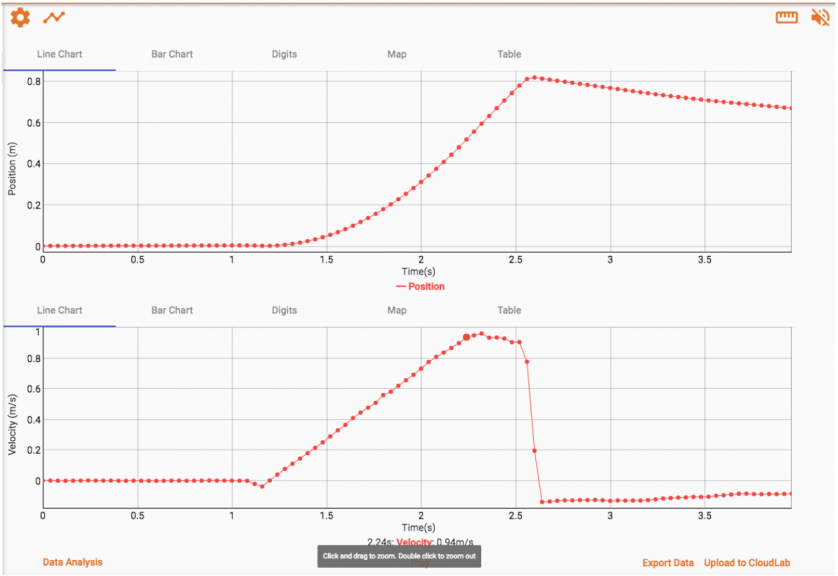Run 3

 Independent Variable
 Mass of Cart 0.258 kg
 Run 3 Observations   Similar to Run 2, the added mass did not affect the velocity of the cart at any point. The GPE and KE of the cart increased as the mass increased compared with Runs 1 and 2. The resultant data is the average KE of the cart at the bottom of the ramp.   Resultant for Data Visualization: KE at bottom of ramp = 0.111 J

Run 3 Trial 3 (Velocity at bottom of ramp = 0.95 m/s)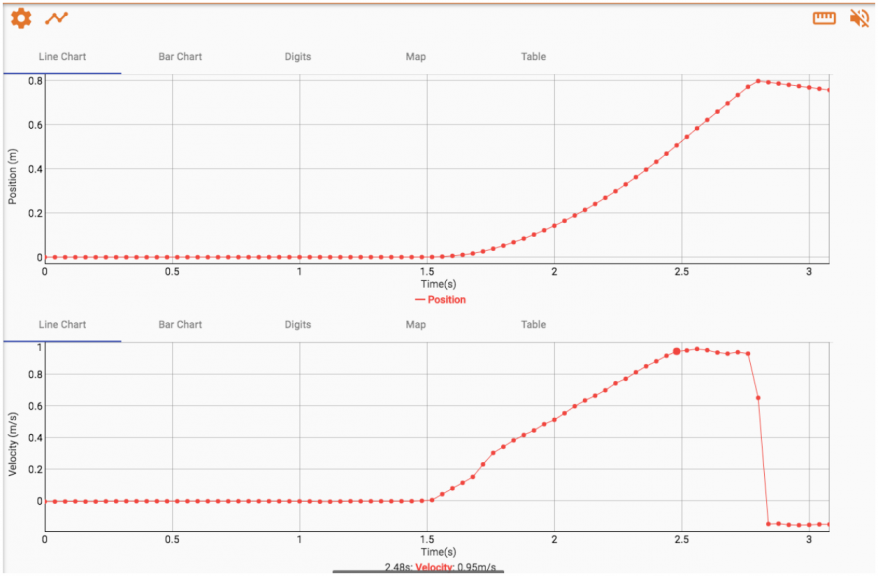#### Data Analysis

We averaged our results across all trials for each run. The results are in our included data tables. When looking at the average data for Run 1, we determined that adding the GPE and KE at every location did not give us the Total Energy in the system. Data was missing. The total energy should stay the same throughout the cart's trip down the ramp because energy does not simply disappear. It can change form but not be destroyed. This means some of the GPE was not just transferring to KE. Some was also transferred to another type of energy.

Looking back at our Key Words from the beginning of the lab, we determined some of the energy was transferring to Thermal Energy through the process of Friction. By subtracting the GPE and the KE from what should be the Total Energy at each location we were able to determine an approximate value for the Thermal Energy at each location. We added this to our data tables for each Run average.

We then drew bar graphs breaking down the type of energy at each location for each of the Run averages. We noticed that no matter what, the bar graph for Total Energy was always equal to the sum of the bar graphs for GPE, KE, and Thermal Energy. The values of those bar graphs changed between those three types of energy, but their sum was always the same. When the mass increased in Runs 2 and 3, the Total Energy increased, but the pattern shown in the bar graphs was the same. Increasing mass had no effect on velocity data, however.

 Run 1 Average
 Height of cart (m) 0.12 0.08 0.06 0.03 0 Displacement (m) 0 0.123 0.253 0.737 0.5 Velocity (m/s) 0 0.433 0.65 0.787 0.9 GPE (J) 0.207 0.138 0.103 0.052 0 KE (J) 0 0.017 0.037 0.054 0.071 Therm. Energy (J) 0 0.052 0.067 0.101 0.136 Total Energy 0.207 0.207 0.207 0.207 0.207

 Run 2 Average
 Height of cart (m) 0.12 0.08 0.06 0.03 0 Displacement (m) 0 0.123 0.253 0.377 0.503 Velocity (m/s) 0 0.453 0.663 0.813 0.927 GPE (J) 0.253 0.169 0.126 0.063 0 KE (J) 0 0.022 0.047 0.071 0.092 Therm. Energy (J) 0 0.062 0.08 0.119 0.161 Total Energy 0.253 0.253 0.253 0.253 0.253

 Run 3 Average
 Height of cart (m) 0.12 0.08 0.06 0.03 0 Displacement (m) 0 0.123 0.257 0.373 0.5 Velocity (m/s) 0 0.457 0.667 0.81 0.927 GPE (J) 0.303 0.202 0.152 0.076 0 KE (J) 0 0.027 0.057 0.085 0.192 Therm. Energy (J) 0 0.074 0.121 0.142 0.192 Total Energy 0.303 0.303 0.303 0.303 0.303

Resultant Data Visualization: Mass of the cart compared to average Kinetic Energy of the cart at the bottom of the ramp.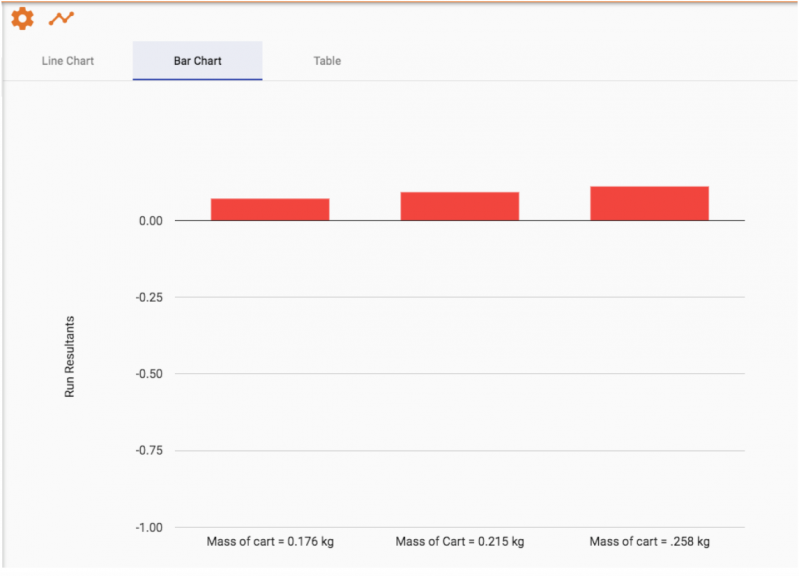#### Conclusion

After examining the data, some parts of our hypothesis from the beginning of the lab are invalid while some parts are valid. We predicted that increasing the mass would increase the velocity of the cart and the KE of the cart. Increasing the mass of the cart had no effect on the velocity of the cart, and while the KE did increase, this was because of the increase in mass, not an increase in velocity, which is what we predicted. We believe the increased mass of the cart had no effect on the velocity because gravity accelerates all objects near Earth's surface at approximately the same rate, regardless of mass. We were correct in predicting that the increase in mass would increase the GPE of the cart because increasing either mass or height increases GPE. We were also correct in predicting that the Total Energy would increase because of the increase in GPE.

TEACHERS: Find the assignable, interactive version of this lesson in PocketLab Notebook here.#### Society for Science Awards Teachers Hundreds of Thousands of Dollars#### Potential Energy to Kinetic Energy Experiment: Gravity | PocketLab